# How to Create simulated data for classification in Python?

• Last Updated : 17 Oct, 2021

In this article, we are going to see how to create simulated data for classification in Python.

We will use the sklearn library that provides various generators for simulating classification data.

Attention reader! Don’t stop learning now. Get hold of all the important Machine Learning Concepts with the Machine Learning Foundation Course at a student-friendly price and become industry ready.

## Single Label Classification

Here we are going to see single-label classification, for this we will use some visualization techniques.

### Example 1: Using make_circles()

make_circles generates 2d binary classification data with a spherical decision boundary.

## Python3

 `from` `sklearn.datasets ``import` `make_circles``import` `pandas as pd``import` `matplotlib.pyplot as plt`` ` `X, y ``=` `make_circles(n_samples``=``200``, shuffle``=``True``, ``                    ``noise``=``0.1``, random_state``=``42``)``plt.scatter(X[:, ``0``], X[:, ``1``], c``=``y)``plt.show()`

Output: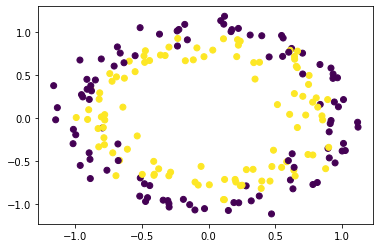### Example 2: Using make_moons()

make_moons() generates 2d binary classification data in the shape of two interleaving half circles.

## Python3

 `from` `sklearn.datasets ``import` `make_moons``import` `pandas as pd``import` `matplotlib.pyplot as plt`` ` `X, y ``=` `make_moons(n_samples``=``200``, shuffle``=``True``,``                  ``noise``=``0.15``, random_state``=``42``)``plt.scatter(X[:, ``0``], X[:, ``1``], c``=``y)``plt.show()`

Output: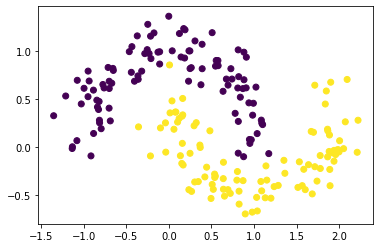### Example 3. Using make_blobs()

make_blobs() generates data in form of blobs that can be used for clustering

## Python3

 `from` `sklearn.datasets ``import` `make_blobs``import` `pandas as pd``import` `matplotlib.pyplot as plt`` ` `X, y ``=` `make_blobs(n_samples``=``200``, n_features``=``2``, centers``=``3``,``                  ``shuffle``=``True``, random_state``=``42``)``plt.scatter(X[:, ``0``], X[:, ``1``], c``=``y)``plt.show()`

Output: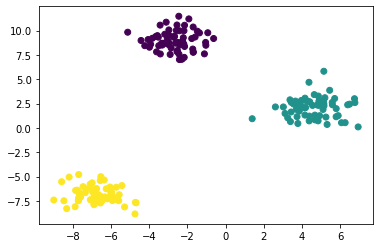### Example 4. Using make_classification()

make_classification() generates a random n-class classification problem

## Python3

 `from` `sklearn.datasets ``import` `make_classification``import` `pandas as pd``import` `matplotlib.pyplot as plt`` ` `X, y ``=` `make_classification(n_samples``=``100``, n_features``=``5``,``                           ``n_classes``=``2``,``                           ``n_informative``=``2``, n_redundant``=``2``,``                           ``n_repeated``=``0``,``                           ``shuffle``=``True``, random_state``=``42``)``pd.concat([pd.DataFrame(X), pd.DataFrame(``    ``y, columns``=``[``'Label'``])], axis``=``1``)`

Output: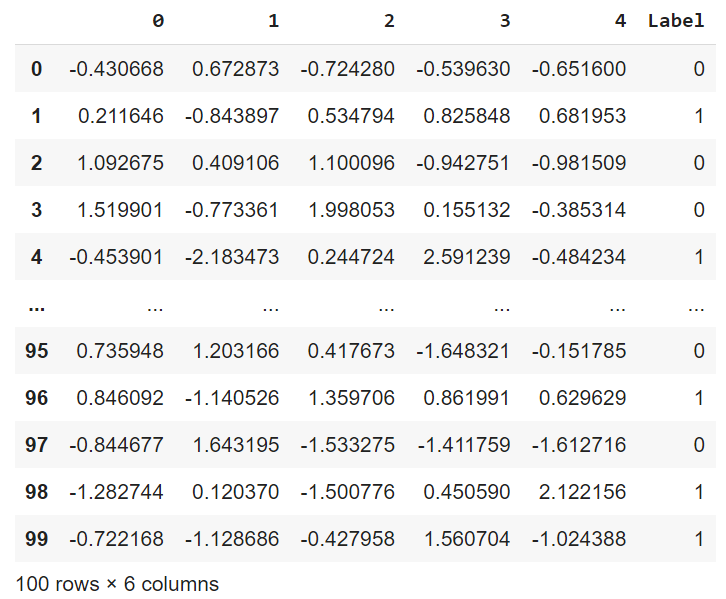## Multi-Label Classification

make_multilabel_classification() generates a random multi-label classification problem.

## Python3

 `from` `sklearn.datasets ``import` `make_multilabel_classification``import` `pandas as pd``import` `matplotlib.pyplot as plt`` ` `X, y ``=` `make_multilabel_classification(n_samples``=``100``, n_features``=``5``, ``                                      ``n_classes``=``2``, n_labels``=``1``,``                                      ``allow_unlabeled``=``False``,``                                      ``random_state``=``42``)``pd.concat([pd.DataFrame(X), pd.DataFrame(y, ``                                         ``columns``=``[``'L1'``, ``'L2'``])],``          ``axis``=``1``)`

Output: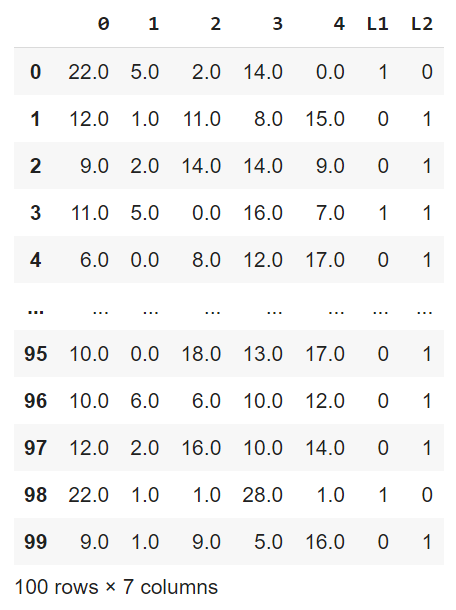My Personal Notes arrow_drop_up